• Option Explicit Sub test() Dim i As Long For i = 2 To Sheet1.Range("A1000000").End(xlUp).Row Sheet1.Cells(i, 2) = GetStr(Sheet1.Cells(i, 1).Value, "^.*[a-zA-Z](?=\d)") ...
Option Explicit

Sub test()
Dim i As Long

For i = 2 To Sheet1.Range("A1000000").End(xlUp).Row
Sheet1.Cells(i, 2) = GetStr(Sheet1.Cells(i, 1).Value, "^.*[a-zA-Z](?=\d)")
Sheet1.Cells(i, 3) = GetStr(Sheet1.Cells(i, 1).Value, "\d{3}[A-Z]+\$")

Next
End Sub

Function GetStr(str As String, pattern As String) As String
Dim reg As Object, mc
Set reg = CreateObject("VBScript.RegExp")
reg.pattern = pattern
Set mc = reg.Execute(str)
If mc.Count > 0 Then
GetStr = mc.Item(0).Value
Else
GetStr = ""
End If

End Function

展开全文• 12, excel vba 常用字符串处理函数

万次阅读 多人点赞 2018-09-10 23:24:51
处理excel数据 很多时候要和字符串打交道, 比如 0,想要知道一个字符串的长度是多少? 1, 判断字符串是不是含有你需要的字符 2, 截取字符串的某一个部分 3, 将字符串里的某个字符进行替换 4, 把字符串按某个字符...

处理excel数据 很多时候要和字符串打交道, 比如

0,想要知道一个字符串的长度是多少?

1, 判断字符串是不是含有你需要的字符

2, 截取字符串的某一个部分

3, 将字符串里的某个字符进行替换

4, 把字符串按某个字符分割出来

接下来一一介始这几个函数,

0,用 len() 函数获得一个字符串长度

Public Sub  点点didi12()

my_string = "diandian didi"
str_len = Len(my_string)
Debug.Print str_len

End Sub

运行一个,就可以在 立即窗口中看到长度值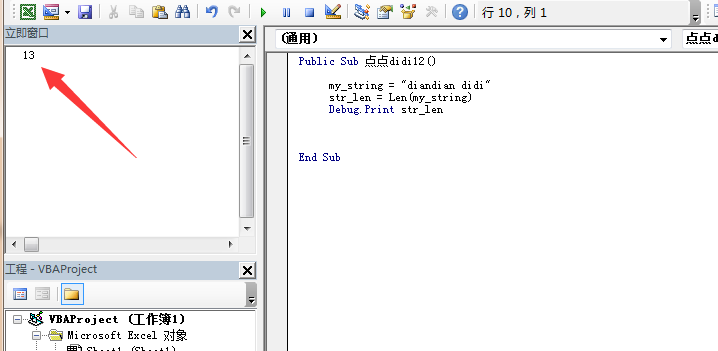1, 使用 instr() 判断是不是含某个字符,如果有,就会返回该字符出现的位置

Public Sub diandiandidi12()

my_string = "diandian didi"
my_exp = "an"

Index = InStr(my_string, my_exp)

If Index > 0 Then
Debug.Print Index

Else
Debug.Print "sorry ,no find you exp char"

End If

End Sub

如果字符串中有你需要的字符, 就会返回该字符第一次出现的位置, 如果没有,就说下sorry.运行结果如下, 你也可以复制该代码,改下 my_exp的值试试看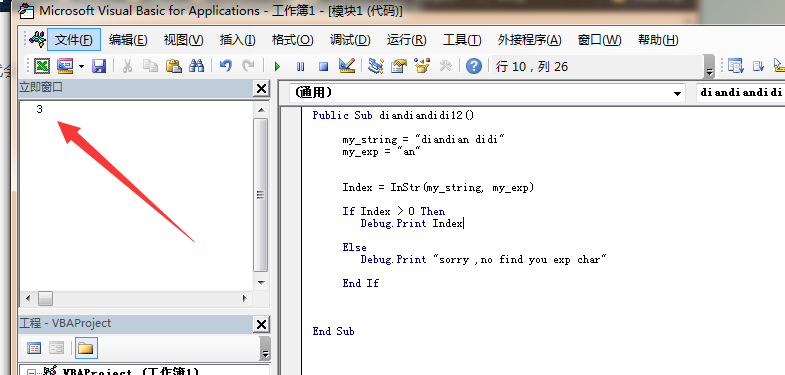2 ,使用 left()  mid() ,right() 截取字符串.

left是左截取, 从字符串左边开始,截取你需要的字符个数, right则从右边开始. mid的话,需要你输入字符串截取的位置和函数

接下来给大家演示下这三个函数

Public Sub diandiandidi12()

my_string = "start diandian didi end"

start_index = 3
jiequ_len = 5

left_char = Left(my_string, jiequ_len)
Debug.Print "left 5 char: " & left_char

right_char = Right(my_string, jiequ_len)

Debug.Print "right  5 char: " & right_char

mid_char = Mid(my_string, start_index, jiequ_len)
Debug.Print "mid_char  5 char: " & mid_char

End Sub
运行结果如下: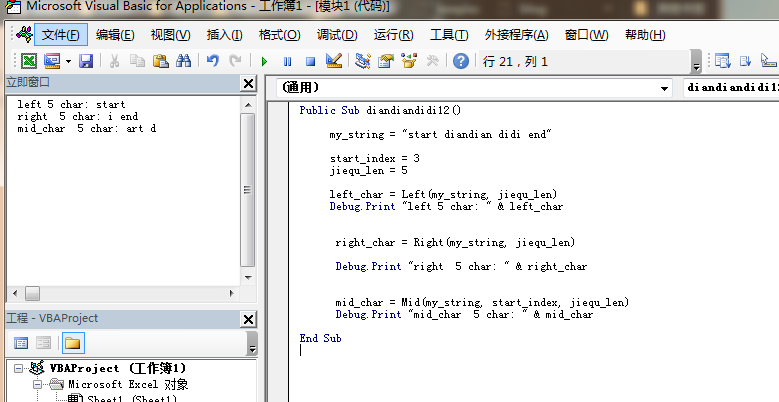3 ,使用 replace() 把字符串中的某个字符替换成其它字符. 接下来把字符串中的an 替换成love

Public Sub diandiandidi12()

my_string = "start diandian didi end"

find_char = "an"
replace_char = "love"

replace_result = Replace(my_string, find_char, replace_char)

Debug.Print replace_result

End Sub

运行结果: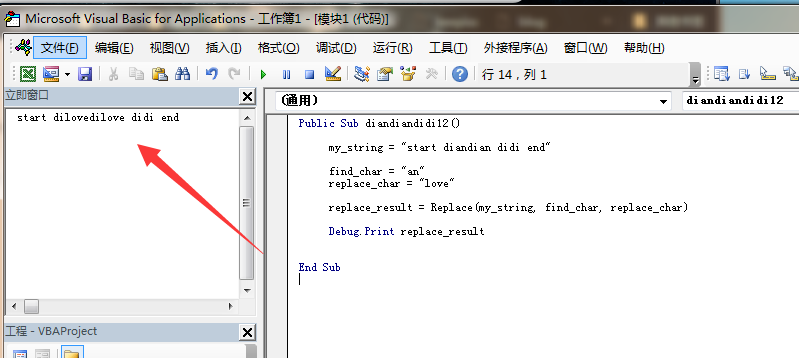4 ,使用 split() 把字符串按某个字符分割, 接下来把字符串按 an字符进行分割

Public Sub diandiandidi12()

my_string = "startdiandiandidiend"

split_char = "an"

split_results = Split(my_string, split_char, -1)

For Each result In split_results

Debug.Print result

Next

End Sub

运行后如下: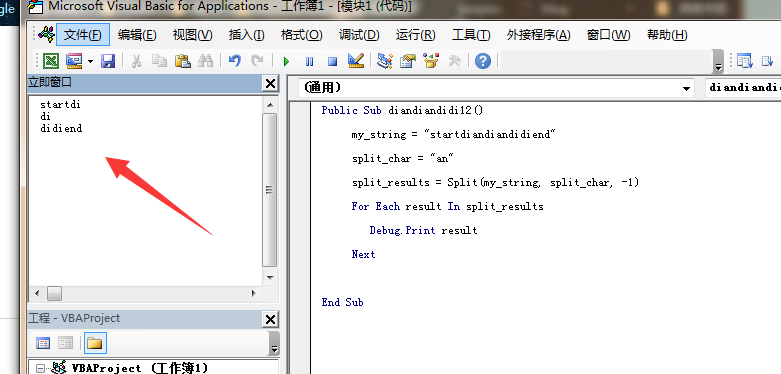split_results = Split(my_string, split_char, -1)  这句中, 最后面的 -1 ,表示返回所有的分割结果, 这里看到,返回了三个分割的结果.

这个分割函数执行后,返回的是一个数组, 也就是split_results是一个数组, 数组是一种数据结构, 你可以把这种结构想像成一排柜子,每个柜子都有一个序号, 从0开始进行标号,每个柜子里都可以放一些东西, 在这里的话, 可以画草图给大家做个理解: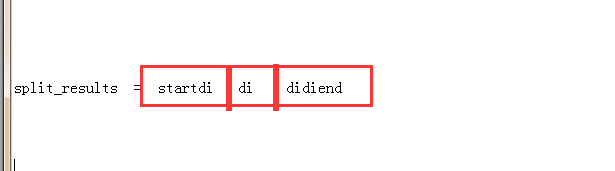split_results可以看成有三个柜子组成的数组, 如果你想得到里面的某个数据, 可能用 数组名(index) 的方式, 比如你想得到第一个数据  , 就可以用   split_results(0)    , 哦, 这里的序号是从0开始的. 0 到1到2...

Public Sub diandiandidi12()

my_string = "startdiandiandidiend"

split_char = "an"

split_results = Split(my_string, split_char, -1)

Debug.Print "the first split result is : " & split_results(0)

End Sub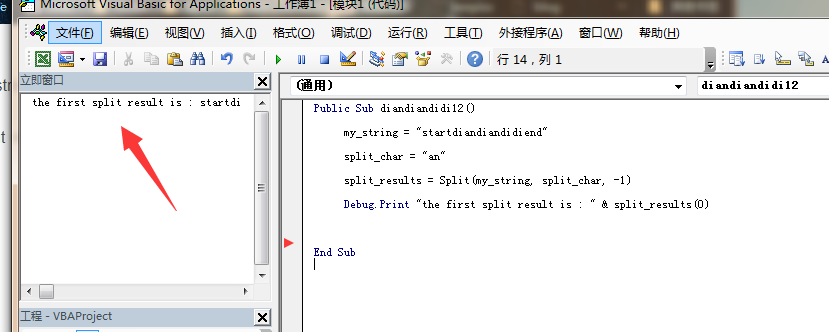常用函数的话还有 trim()  ,可以把字符串前后的空字符串去掉, 如果你想了解更多函数,就需要靠你自己百度or 谷歌啦.

展开全文• 使用split() 处理excel里的文本字符串—转为array 使用splint()处理数据时，不受 option base 影响 Sub test2001() Rem range("f1").value="1001|1,2005|1,1006|1,2009|1,2006|1,4001|60" arr1 = Split(Range(...

使用split() 处理excel里的文本字符串—转为array

使用splint()处理数据时，不受 option base 影响

Sub test2001()

Rem range("f1").value="1001|1,2005|1,1006|1,2009|1,2006|1,4001|60"

arr1 = Split(Range("f1"), ",")

For i = 0 To UBound(arr1)
Debug.Print arr1(i)
Next i

End Sub

转2维数组

拆解2维数组的例子—没搞定

Sub testarr1()

Rem 把一个字符串切割两次成为1个2维数组好像很难？
Rem 数据1：张三1|男，李四1|女，张三2|男，李四2|女，张三3|男，李四3|女，张三4|男，李四4|女
Rem 数据2：张三1(男)，李四1(女)，张三2(男)，李四2(女)，张三3(男)，李四3(女)，张三4(男)，李四4(女)

Dim arr1
Dim arr2

arr1 = Split(Range("F1"), "，")

For i = 1 To UBound(arr1)
ReDim arr2(1 To i, 1 To 2)
arr2(i, 1) = Split(arr1(i), "|")
'   arr2(i, 1) = Application.WorksheetFunction.Mid(arr1(i).Value, 1, 3) '用Application.Left 居然不行
'   arr2(i, 2) = Application.WorksheetFunction.Mid(arr1(i).Value, 5, 1)
Next

For i = 1 To UBound(arr1)
For j = 1 To 2
Debug.Print arr2(i, j)
Next j
Next i

End Sub

第2个方法好了点?

Sub testarr1()

Rem 把一个字符串切割两次成为1个2维数组好像很难？
Rem 数据1：张三1|男，李四1|女，张三2|男，李四2|女，张三3|男，李四3|女，张三4|男，李四4|女
Rem 数据2：张三1(男)，李四1(女)，张三2(男)，李四2(女)，张三3(男)，李四3(女)，张三4(男)，李四4(女)

Dim arr1
Dim arr2

arr1 = Split(Range("F1"), "，")

For i = 1 To UBound(arr1)
ReDim arr2(1 To i, 1 To 2)
arr2(i, 1) = Left(arr1(i), 3) '用Application.Left 居然不行
arr2(i, 2) = Right(arr1(i), 1)
Next

For i = 1 To UBound(arr1)
For j = 1 To 2
Debug.Print arr2(i, j)
Next j
Next i

End Sub

展开全文• 用VBA提取字符串中的数字

千次阅读 2021-04-26 15:15:28
上一篇讲了用 python 提取字符串中的数字，这次用VBA来事项。总体思路和 python 是一样的，代码如下： Option Explicit Sub GetNumbers() Dim from_string As String, convert_numbers As String Dim i, j, k, ...

上一篇讲了用 python 提取字符串中的数字，这次用VBA来实现。总体思路和 python 是一样的，代码如下：

Option Explicit

Sub GetNumbers()

Dim from_string As String, convert_numbers As String
Dim i, j, k, m, first_number_location
Dim i1 As String
Dim check_start(9) As String, check_end(10) As String
Dim split_string() As String, get_numbers() As String

' 给 from_string 赋值
from_string = Cells(1, 1)
from_string = CStr(from_string)

' 先求出 check_start 和 check_end
' 用于后续检验 from_string 中每个字符是否是数字
For i = 0 To 9
i1 = CStr(i)
check_start(i) = i1
check_end(i) = i1
Next i

check_end(10) = "."

' 将 from_string 拆分，每个字符都存到 split_string 中
ReDim split_string(Len(from_string) - 1)
For i = 1 To Len(from_string)
split_string(i - 1) = Mid(from_string, i, 1)
Next i

' 先求出 split_string 中第一个数字及其位置
For i = LBound(split_string()) To UBound(split_string())
For j = LBound(check_start()) To UBound(check_start())
If split_string(i) = check_start(j) Then
ReDim get_numbers(UBound(split_string) - i)
get_numbers(0) = split_string(i)
first_number_location = i
End If
Next j
Next i

m = 1

' 从第一个数字开始，求出之后紧连的每个数字，包括小数点
For j = first_number_location + 1 To UBound(split_string())
For k = LBound(check_end()) To UBound(check_end())
If split_string(j) = check_end(k) Then
get_numbers(m) = split_string(j)
m = m + 1
End If
Next k
Next j

' 把 get_numbers() 输出
For j = LBound(get_numbers()) To UBound(get_numbers())
convert_numbers = convert_numbers & get_numbers(j)
Next j

MsgBox convert_numbers

End Sub

展开全文• 无论是EXCEL的操作，还是VBA的操作，都会对大家有所帮助。知识的讲解过程中，希望大家得到哪怕是一点点的收获，我的时间就是有意义的。今日给大家讲VBA的循环，这个是非常基础的知识，在VBA的相关内容中我也一直在用...vba遍历字符串
• Private Sub Test() Dim sourceStr As String sourceStr = "1234567891234567891" Dim length As Integer length = Len(sourceStr) Dim ary() As String ary = SplitToLine(sour...VBA 字符串处理
• vba 根据字节数截取字符串 'str 要截取的字符串 'byteLen 需要截取的字节长度 ' return byteLen长度的字符串 Public Function kiritoruStr(str As String，byteLen as Integer) As String Dim chA As String ...字符串 vba
• 功能：根据空格，把单元格6的字符串拆分到7，8，9单元格。 在execl中，按alt+f11打开vba代码输入框，复制粘贴本代码到里面，然后执行。 使用到的知识点 1.vba的循环 2.单元格内容的读取和写入。vba excel
• 使用regexp对象那个已经设置好的表达式（pattern属性）在字符窜s中查找符合t条件的文本 03 替换 regexp.replace(s,r)并未修改原文本是，而是将替换后的节骨作为一个新的字符窜 cells（i，2）=...
• 例如excel中某一列直接粘贴做为查询参数，需要将该字符串已换行符进行拆分转为数组 let strArr = str.split(/[(\r\n)\r\n]+/) strArr .forEach((item,index)=>{ if(!item){ //删除结尾为空的元素 snsArr....
• strText为需要处理的字符串，共有有8个字符，经过正则替换，结果保存在变量strNew中，不难看出首位各有一个空格，并且每个字符之间也插入了一个空格。这是如何实现的呢？ 正则对象不指定匹配模式时，相当于Pattern =...正则 正则表达式 字符串转数组 匹配模式 Pattern
• 上次我介绍了有规律字符串的提取方法，使用excel自带函数RIGHT()和LEN()实现。但是对于要提取的字符串如果我们不能确定其字符数， 那么我们使用这种方式就无能为力。如下要提取H列里每个单元格课程的名称，课程名称...vba 字符串 excel
• 下载源文件 ： http://lwl0606.cmszs.com/archives/excel-vba-string-function.html在Excel 里面 如果要合并字符串可以用函数 =CONCATENATE(A4,B4,C4) 当然也可以 =A4 & B4 & C4 下面的函数可以实现分组...
• VB分割字符串为数组 字符串转数组  VB分割字符串为数组，VB字符串转数组： Dim B() As String B() = Split(“1,2,3,4,dsd-ad5,6,45,656465,564,45″, “,”) ‘分割为数组 MsgBox B(4) ‘读取第五个，从0开始 ...
• 详细介绍了vba字符串的处理，非常实用 vba是现在办公必不可少的计算机语言vba
• 用 Excel 处理数据时，有时需要对字符串进行拆分。对于比较简单的拆分，使用 Excel 函数可以顺利完成，但碰到一些特殊需求，或者拆分的规则比较复杂时，则很难用 Excel 实现了。这里列出一些拆分需求示例，分析拆分...spl
• EXCEL VBA字符串替换

万次阅读 2015-03-30 17:00:52
修正规则如下，将原来的连字符形式改成驼峰式。 原始字段 修正后字段 session_id sessionId 打开VBA这里是使用excel自带的VBA进行处理。 在excel里调出VBA的方法如下图所示。在某个表单上右击，并点击”查看...vba 表单
• Dim d As Object Set d = CreateObject("scripting.dictionary") Dim r as Integer Dim arr r = Cells(Rows.Count, 1).End(xlUp).Row arr = Range("a1:o" & r) '省略一些，理解思路即可 d(arr(i, 2)) = d(arr(i,...
• 以下为代码，采用textbox空间获得输入字符的长度，并通过button_click触发 设置了判断功能，判断当前截取的字符元素长度是否与采集长度一致，如果不一致则舍弃该采集结果，避免存储重复的数据 Sub button_click() ...
• 实现标题的功能，方法也很多。...如有字符串： string str = "Insus.NET"; 方法一： public void SplitString(string str) { int len = str.Length; for (int i = 0; i &lt; l...
• 需求：手头有个几千行的函数列表，每个函数名都采用驼峰式命名方式命名，如...为了分析各函数的分布，需要将函数名拆分成以单词词组。代码：Sub CamelCase() Dim rCell As Range Dim lCount As Long Wi...
• excel中用宏拆分字符串

千次阅读 2009-10-30 22:38:00
晚上有个朋友打电话问我可不可以给他做个小程序，要实现的功能比较简单，想把"xxx省xxx市xxx区(县)"这类的字符串拆分成省市区三段，由于原本数据就是在excel中存放的，想了想应该可以通过VBA来实现，虽然没搞过，但...excel string security vba google
• 1.待分割字符串: //分别间隔一个空格,两个空格,一个tab制表符 String str = "a b c d"; 字符串中的的空白分别为：单个空格，两个空格，tab制表符。 2. 四种分隔符比较 //四种分隔符 String[] arr1 = str.split...java 字符串
• 题目很简单，实现字符串的切割与反转，并且写入文件。 方法如下: public static void Flip_string(String filePath,String s) throws Exception { PrintWriter pw=new PrintWriter(filePath); ...
• 由Regex提供支持的自定义字符串类，可进行搜索，替换，拆分等操作 目的是通过添加字符串类来简化字符串的使用，该类类似于完全面向对象的语言中的字符串字符串类：在将该库引用到您的项目后，可以像下面这样...
• 字符串切分操作中忽略引号中的分隔符（具体函数实现） 在数据分析当中常常会遇到字符串分割操作，但是有时不想分割引号中的分割符，不限于引号，这时候单靠 split 函数是无能为力的，我这写了Scala以及Python的递归...字符串切分
• 1、split(待拆分字符,分隔符字符) 将字符串按指定分隔符拆分为多个子串， 需用数组接受结果集（动态数组）; 再用for 循环将每个元素赋值给单元格 例如： dim a() as string,i% a=split(s,&amp;quot;,...提高 vba...